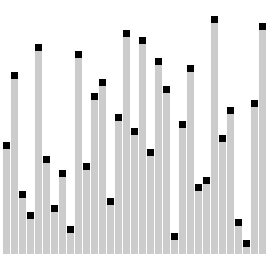# Python Example – Write a Python program to sort a list of elements using Comb sort# (Python Example for Citizen Data Scientist & Business Analyst)

Write a Python program to sort a list of elements using Comb sort.

The Comb Sort is a variant of the Bubble Sort. Like the Shell sort, the Comb Sort increases the gap used in comparisons and exchanges. Some implementations use the insertion sort once the gap is less than a certain amount. The basic idea is to eliminate turtles, or small values near the end of the list, since in a bubble sort these slow the sorting down tremendously. Rabbits, large values around the beginning of the list do not pose a problem in bubble sort. In bubble sort, when any two elements are compared, they always have a gap of 1. The basic idea of comb sort is that the gap can be much more than 1.

Visualization of comb sort:Animation credits : Jerejesse

Sample Solution:

Python Code:

``````
def comb_sort(nums):
shrink_fact = 1.3
gaps = len(nums)
swapped = True
i = 0

while gaps > 1 or swapped:
gaps = int(float(gaps) / shrink_fact)

swapped = False
i = 0

while gaps + i < len(nums):
if nums[i] > nums[i+gaps]:
nums[i], nums[i+gaps] = nums[i+gaps], nums[i]
swapped = True
i += 1
return nums

num1 = input('Input comma separated numbers:n').strip()
nums = [int(item) for item in num1.split(',')]
print(comb_sort(nums))
``````

Sample Output:

```Input comma separated numbers:
5, 15, 37, 25, 79
[5, 15, 25, 37, 79]```

## Write a Python program to sort a list of elements using Comb sort

#### Free Machine Learning & Data Science Coding Tutorials in Python & R for Beginners. Subscribe @ Western Australian Center for Applied Machine Learning & Data Science.```